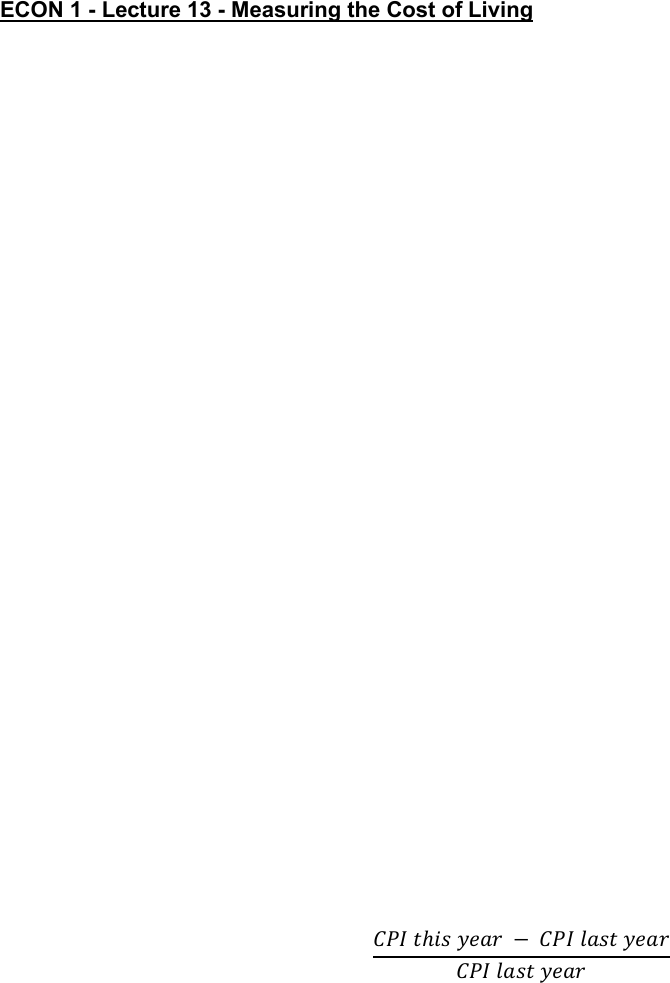Class Notes (1,100,000)
US (460,000)
UC-Irvine (10,000)
ECON (1,000)
ECON 1 (50)
Lecture 13

# ECON 1 Lecture Notes - Lecture 13: Gdp Deflator, Interest Rate, Nominal Interest RatePremium

Department
Economics
Course Code
ECON 1
Professor
SARRAF, G
Lecture
13

This preview shows half of the first page. to view the full 3 pages of the document.ECON 1 - Lecture 13 - Measuring the Cost of Living
Look for the answers to these questions
What is the Consumer Price Index (CPI)?
How is it calculated? What is it used for?
What are the problems with the CPI? How serious are they?
How does the CPI differ from the GDP deflator?
How can we use the CPI to compare dollar amounts from different years? Why would we
want to do this anyway?
How can we correct interest rates for inflation?
The Consumer Price Index
Consumer price index (CPI)
Measure of the overall level of prices
Measure of the overall cost of goods and services
Brought by a typical consumer
Computed and reported every month by the Bureau of Labor Statistics
Calculating CPI
The Bureau of Labor Statistics (BLS) surveys consumers to determine what’s in
2. Find the prices
The BLS collects data on the prices of all the goods in the basket
Use the prices to compute the total cost of the basket
4. Chose a base year and compute the CPI
Cost of basket of goods and services in current year divided by cost of basket in
base year
Times 100
5. Compute the inflation rate
The percentage change in the CPI from the preceding period
𝐼𝑛𝑓𝑙𝑎𝑡𝑖𝑜𝑛 𝑟𝑎𝑡𝑒 = 𝐶𝑃𝐼 𝑡𝑖𝑠 𝑦𝑒𝑎𝑟 𝐶𝑃𝐼 𝑙𝑎𝑠𝑡 𝑦𝑒𝑎𝑟
𝐶𝑃𝐼 𝑙𝑎𝑠𝑡 𝑦𝑒𝑎𝑟 ×100
Problems with the CPI
Substitution Bias
Over time, some prices rise faster than others
Consumers substitute toward goods that become relatively cheaper, mitigating
the effects of price increases
The CPI misses this substitution because it uses a fixed basket of goods
Thus, the CPI overstates increases in the cost of living
Introduction of New Goods